Jarvis' Blog (总有美丽的风景让人流连) 总有美丽的风景让人流连

# 半监督学习(Semi-Supervised Learning, SSL)

2021-08-07
Jarvis
Post

## 1. Introduction

### 1.1 半监督学习

SSL 介于监督学习和无监督学习之间, 数据集 $$X = \{x_i\}$$ 可以分为两部分, 有标签的数据集 $$X_l = \{(x_1, y_1), \dots, (x_l, y_l)\}$$ 和无标签数据集 $$X_u = \{x_{l+1}, \dots, x_{l+u}\}$$. 我们可以从两个角度来看待半监督学习:

• 我们使用 $$X_l$$ 来确定分类边界, 并期望使用 $$X_u$$ 来更好地估计数据分布 $$p(x)$$, 从而更准确的确定分类边界. 如图 1 所示, 我们可以通过大量的无标签数据寻找低密度区域, 以更好地确定分类边界. 这类方法是比较经典的做法.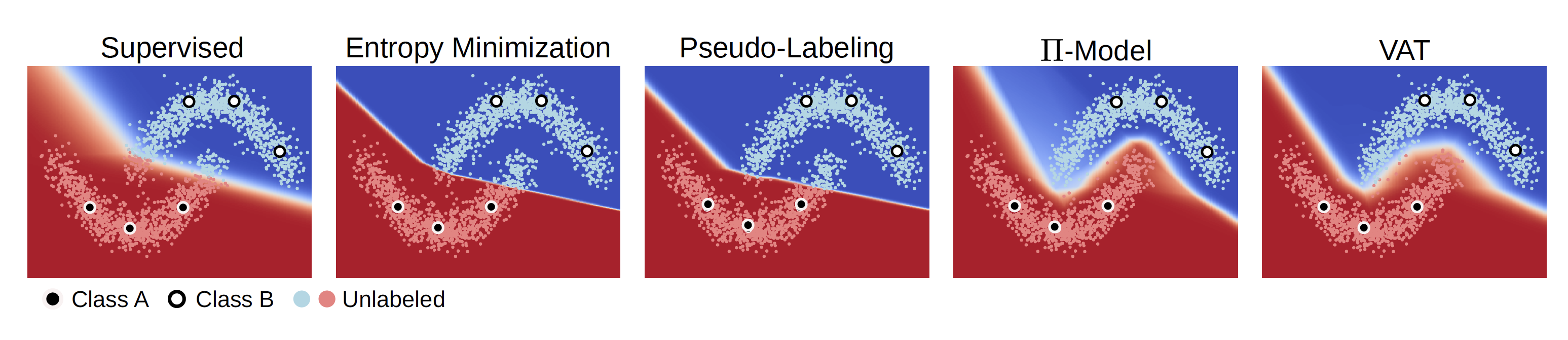• 我们首先使用 $$X_u$$ 基于自监督方法训练一个大模型, 然后使用 $$X_l$$ 对模型进行 finetune, 最后可以进一步蒸馏得到小模型以减少参数量1. 这类方法是在自监督方法有了突破式的发展之后出现的.

### 1.2 分类

SSL 可以大致分类以下几类:

1 Consistency Regularization 一致性约束方法. 数据扰动后, 特征/预测结果不应有显著变化. 从而通过约束扰动前后的数据对应的特征来训练模型.
2 Entropy Minimization 熵极小化. 鼓励模型预测高置信度的结果.
2 Proxy-Label Method 伪标签方法. 通过一个预训练模型对无标签数据打标签, 然后进一步利用打了伪标签的数据训练模型.
3 Generative Models 生成式模型. 结合 GAN 或 VAE, 从无标签数据中学习好的用于分类的判别器.
4 Graph-Based Methods 基于图的方法. 有标签数据和无标签数据可以看做图的节点, 目标是通过节点之间的相似性把有标签节点的标签传播到无标签节点.

• 归纳学习 (Inductive Learning): 学习一个分类器, 从而可以泛化到 unseen 的测试数据集上.
• 直推式学习 (Transductive Learning): 在训练时同时利用测试数据以提高测试数据上的性能, 这种方法不要求泛化到新的测试数据上.

### 1.3 SSL 的假设

SSL 需要一些假设来保证从大规模无标签数据中学习知识是有效的.

1. 光滑性假设 (Smoothness): 如果高密度区域的两个点 $$x_1$$ 和 $$x_2$$ 距离很近, 那么他们的预测结果 $$y_1$$ 和 $$y_2$$ 也应该很近. 反过来, 如果两个点被低密度区域分离, 那么他们的标签应当不同. 该假设对于分类问题是有用的, 但对于回归问题不然.
2. 聚类假设 (Cluster): 如果两个点在同一个聚类, 那么它们很可能属于同一个类别. (聚类假设也可以看做是低密度分离假设, 即分类边界应当落在低密度区域.) 基于该假设, 我们就可以要求数据在局部扰动时, 其预测分类保持不变.
3. 流形假设 (Manifold): 高维数据分布在一个低维流形上. 由于空间大小随着维度指数增长, 这对于建模来说是灾难性的. 然而有了流形假设, 我们可以寻找低维映射来建模数据.

### 1.4 SSL 与其他任务的联系

• SSL 和无监督域适应 (unsupervised domain adaptation, UDA) 是十分接近的任务, 他们都使用有标签和无标签的数据, 区别在于 SSL 的两类数据属于同一个域, 而 UDA 是源域是有标签数据, 目标域是无标签数据. 同时, 如果我们可以在 UDA 中通过一些方法 (如生成式方法) 把目标域数据变成源域数据, 此时我们就可以把众多 SSL 方法应用到 UDA 方法中.

• SSL 与 learning from noisy labels 的任务有密切联系. 基于伪标签的 SSL 方法势必会遇到伪标签存在大量噪声的问题, 此时 SSL (包括基于伪标签的 UDA 方法) 可以应用 noisy labels 任务的方法.

• SSL 还可以利用自监督学习, 通过自监督学习获得好的 warm-up model; 或在半监督学习的过程中, 引入自监督损失来对模型进行正则化 (一致性约束).

• SSL 不同于弱监督学习 (weakly-supervised learning), 后者所有数据都有标签, 强调的是标签为弱标签. 比如分割任务只提供分类标签, 这与 SSL 的目标是不同的.

## 2. Consistency Regularization

$\hat{x} = x + \underbrace{\sum_{j=1}^d \omega_j\textbf{e}_j^x}_{V_{\omega}}$

$\mathbb{E}_{\omega}[\Vert f_{\theta}(x+V_{\omega}) - f_{\theta}(x)\Vert^2] \approx \mathbb{E}_{\omega}[\Vert \mathbf{J}_x V_{\omega}\Vert^2]$

\begin{align} d_{\text{MSE}}(f_{\theta}(x), f_{\theta}(\hat{x})) &= \frac1{C}\sum_{k=1}^C(f_{\theta}(x)_k - f_{\theta}(\hat{x})_k)^2 \\ d_{\text{KL}}(f_{\theta}(x), f_{\theta}(\hat{x})) &= \frac1{C}\sum_{k=1}^C f_{\theta}(x)_k \log\frac{f_{\theta}(x)_k}{f_{\theta}(\hat{x})_k} \\ d_{\text{JS}}(f_{\theta}(x), f_{\theta}(\hat{x})) &= \frac12 d_{\text{KL}}(f_{\theta}(x)_k, m) + \frac12 d_{\text{KL}}(f_{\theta}(\hat{x})_k, m) \\ \end{align}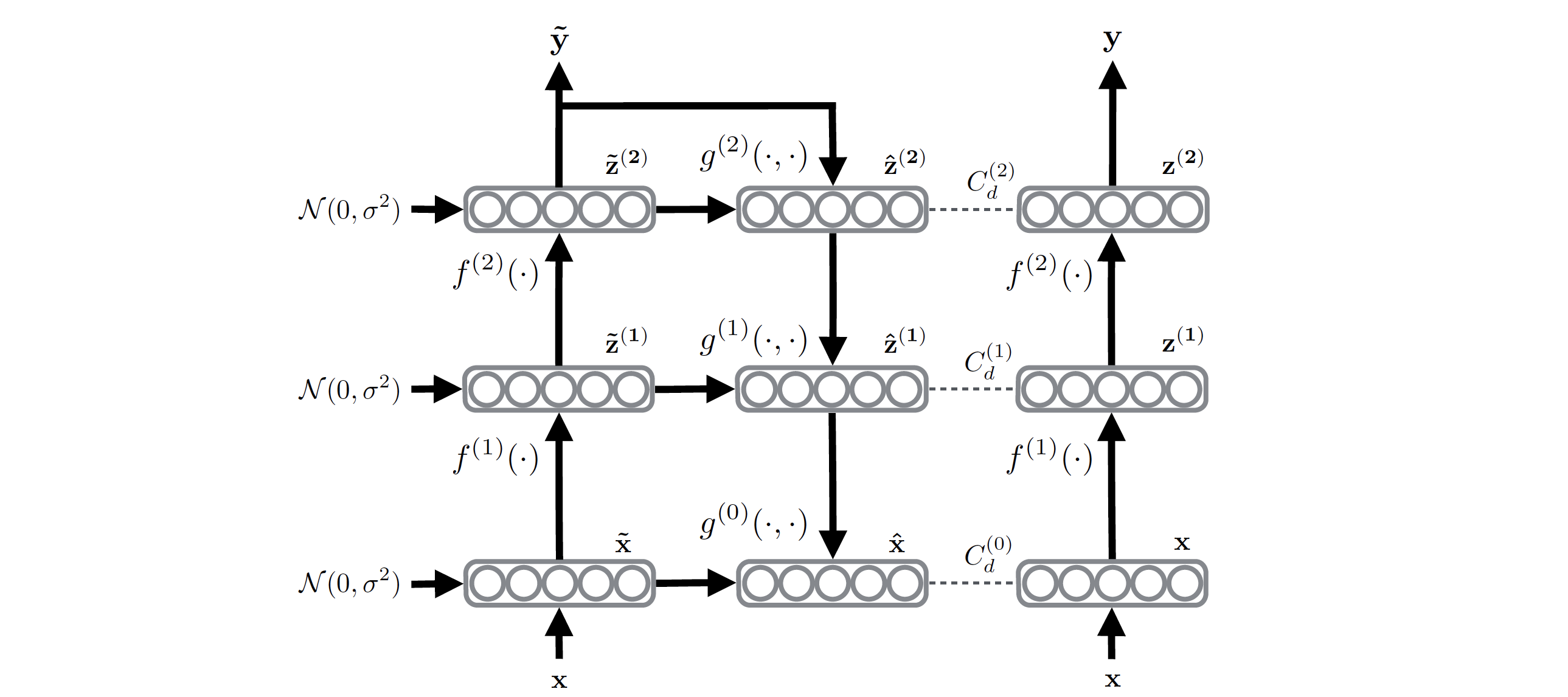Rasmus 等人提出使用 Ladder Network4, 最右侧的 encoder 对 $$x$$ 进行编码, 最左侧的 encoder 在编码过程中加入噪声, 然后通过 decoder 去噪, 同时在每一层的解码输出和右侧 encoder 的编码输出进行一致性约束.

\begin{align} \mathcal{L} &= \mathcal{L}_u + \mathcal{L}_s \\ &= \frac1{\vert D\vert}\sum_{x\in\mathcal{D}}\sum_{l=0}^L\lambda_l d_{\text{MSE}}(z^{(l)}, \hat{z}^{(l)}) + \frac1{\vert\mathcal{D}_l\vert}\sum_{x,t\in\mathcal{D}}H(\tilde{y},t) \end{align}

### 2.2 $$\Pi\text{-Model}$$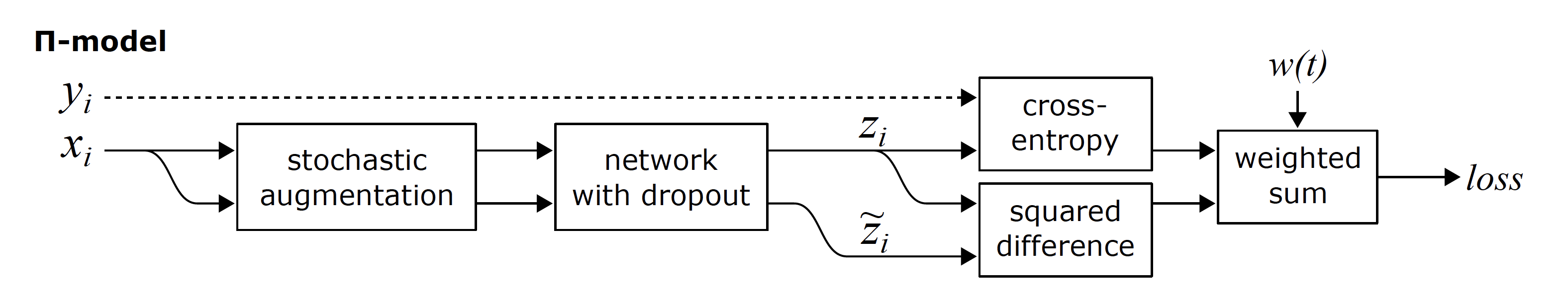Laine 等人提出的 $$\Pi\text{-Model}$$ 5简化了 $$\Gamma\text{-Model}$$, 去除了加噪的 encoder, 只使用一个网络, 利用 augmentation 的输入和 dropout 来实现预测结果的扰动, 并进行约束:

$\mathcal{L} = w\frac1{\vert \mathcal{D}_u\vert}\sum_{x\in\mathcal{D}_u}d_{\text{MSE}}(\tilde{y}_1,\tilde{y}_2) + \frac1{\vert\mathcal{D}_l\vert}\sum_{x,y\in\mathcal{D}_l}H(y, f(x))$

### 2.3 Temporal Ensembling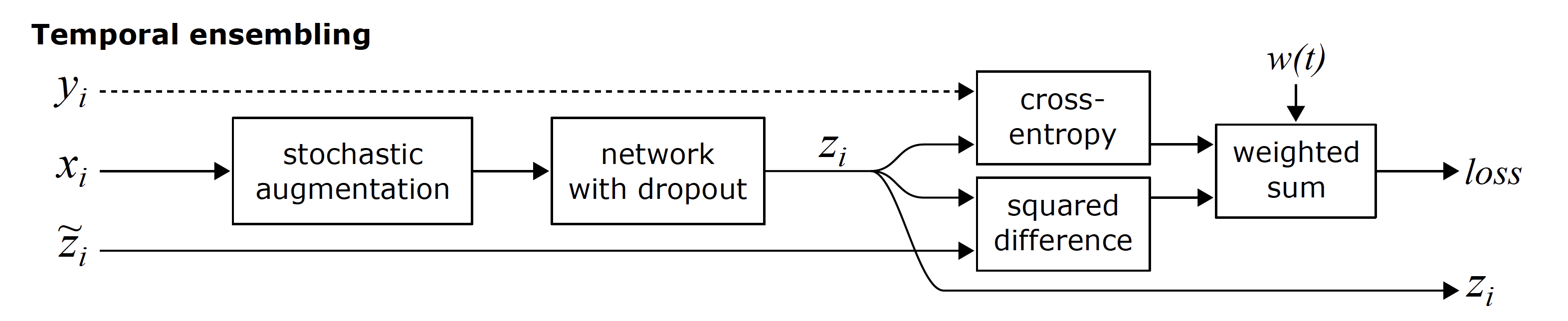$y_{\text{ema}} = \alpha y_{\text{ema}} + (1 - \alpha)\tilde{y}$

$y_{\text{ema}} = (\alpha y_{\text{ema}} + (1 - \alpha)\tilde{y}) / (1 - \alpha^t)$

### 2.4 Mean Teachers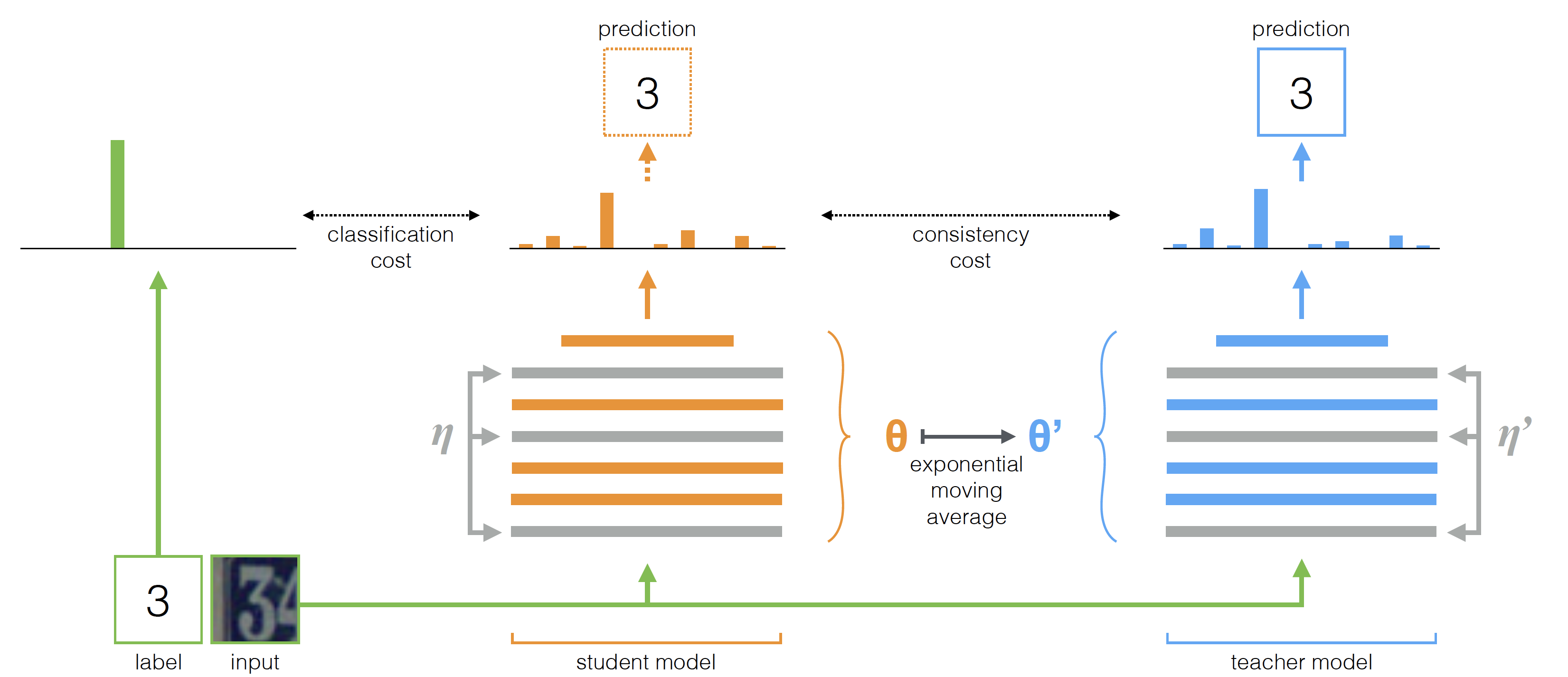Temporal Ensembling 为每一个样本都记录了一个预测结果的 EMA, 但是最新学到的信息也会因为 EMA 模型而被以极其缓慢的速度加入到模型训练的过程中, 每个 epoch 只会更新一次. 同时, 在 $$\Pi\text{-Model}$$ 和 Temporal Ensembling 中, 同一个模型既当老师又当学生, 这样很容易让模型陷入 confirmation bias (即错误被自己放大). 因此, Mean Teacher 方法6被提出来解决这个问题. 与 Temporal Ensembling 不同的是, Mean Teacher 是对模型参数做 EMA, 而非样本的 predictions. 这样的好处是, 每一个 step 模型参数都会更新, 因此 EMA 模型作为 Teacher, 使用梯度更新的模型作为 Student, 可以提供更准确的 targets. 令 $$\theta_t'$$ 是 Teacher Model $$f_{\theta'}$$ 在 step $$t$$ 的参数, 则其更新方法为:

$\theta_t' = \alpha \theta_{t-1}' + (1 - \alpha)\theta_t$

$\mathcal{L} = w\frac1{\vert \mathcal{D}_u\vert}\sum_{x\in\mathcal{D}_u}d_{\text{MSE}}(f_{\theta}(x), f_{\theta'}(x)) + \frac1{\vert\mathcal{D}_l\vert}\sum_{x,y\in\mathcal{D}_l}H(y, f(x))$

### 2.5 Dual Students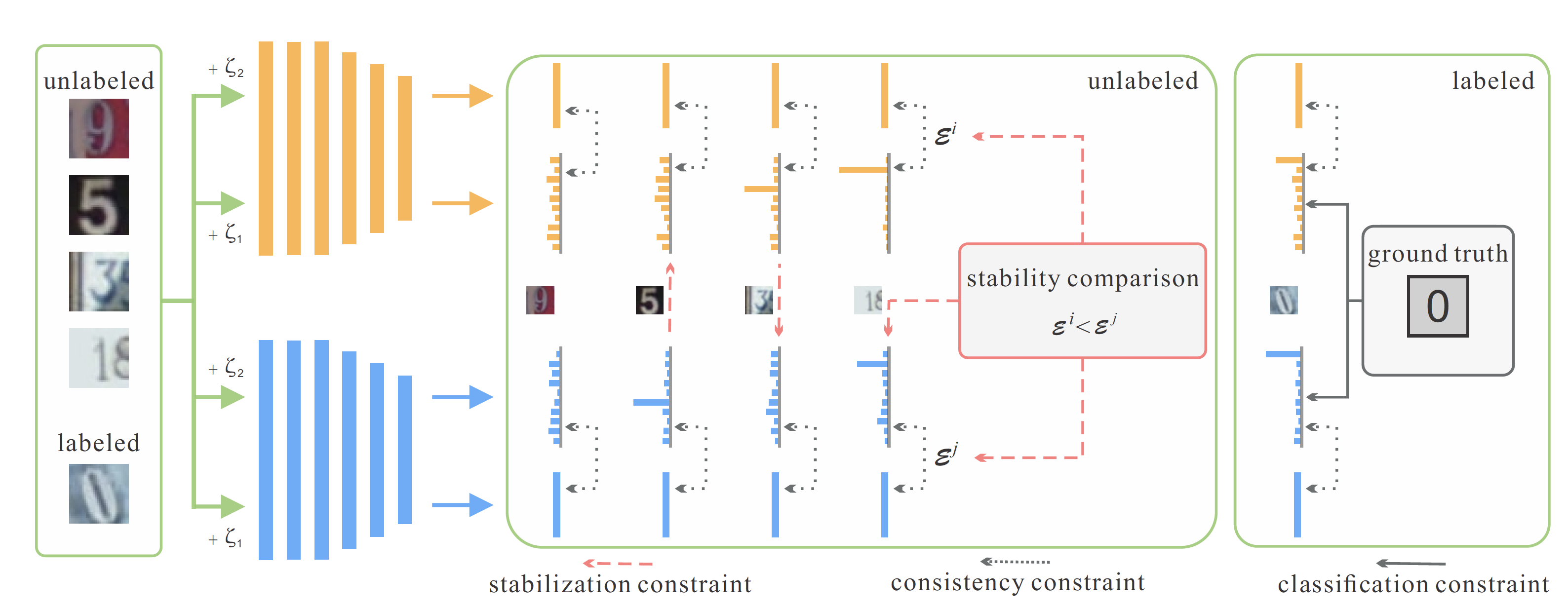Mean Teacher 模型的一个主要的缺点是当训练时间足够长时, Teacher Model 的参数会收敛到 Student Model (假设 Student Model 最后是收敛的), 这样 Teacher 的作用就会随着训练的过程而弱化. Ke 等人提出了 Dual Students7 来解决该问题, 即训练两个独立初始化的模型. 但是, 单纯训练两个独立的模型可能会导致结果相差较大, 而直接施加一致性约束的话又会导致两个模型交换错误的信息而形成模式坍缩变成一个模型, 因此 Dual Students 定义了 stable sample 的概念, 即 $$x$$ 和扰动点 $$\tilde{x}$$ 预测结果相同且离决策边界有一定距离的点. 定义

$\mathcal{E}_i = \Vert f_{\theta^i}(x) - f_{\theta^i}(\tilde{x})\Vert^2$

$\begin{multline} \mathcal{L} = \frac1{\vert\mathcal{D}_l\vert}\sum_{x,y\in\mathcal{D}_l}H(y, f_{\theta^i}(x)) + \lambda_1 \frac1{\vert \mathcal{D}_u\vert}\sum_{x\in\mathcal{D}_u}d_{\text{MSE}}(f_{\theta^i}(x), f_{\theta^i}(\tilde{x})) \\ + \lambda_2 \frac1{\vert \mathcal{D}_u^{\text{ stable for } i}\vert}\sum_{x\in\mathcal{D}_u^{\text{ stable for } i}}d_{\text{MSE}}(f_{\theta^i}(x), f_{\theta^j}(x)) \end{multline}$

## 3. Entropy Minimization

$-\sum_{k=1}^Cf_{\theta}(x)_k\log f_{\theta}(x)_k$

Confidence Regularization

$\sum_{k=1}^Cf_{\theta}(x)_k\log f_{\theta}(x)_k$

$-\sum_{k=1}^C\frac1K\log f_{\theta}(x)_k$

## 4. Proxy-Label Methods

Proxy-Label 方法通常也称为伪标签 (pseudo-label) 方法, 是利用预训练模型对无标签数据打伪标签后, 和有标签数据结合起来训练模型的一类方法. 这类方法的典型实现有 self-training 和 multi-view training.

### 4.1 Self-Training

• Pseudo-label and confirmation bias11
• Pseudo-label and label propagation1213

### 4.2 Multi-View Training

Multi-View Training 的一个代表性的工作是 Co-Training14. 在数据存在两个条件独立的 views 时, 使用两种 views 分别训练两个模型. 比如, 两个 views 可以是 RGB 和 Depth; 图像和文本等. 对于每个无标签数据, 如果在模型 $$j$$ 的预测结果高于阈值 $$\tau$$, 则把它加入模型 $$i$$ 的训练集, 从而实现两个模型相互监督训练.

• Tri-Training15

## 5. Holistic Methods

Holistic 方法是把 SSL 已有的的不同路线的 SOTA 方法集成起来的一类方法, 代表性的工作主要是 Google 一系列的 *Match 方法.

### 5.1 MixMatch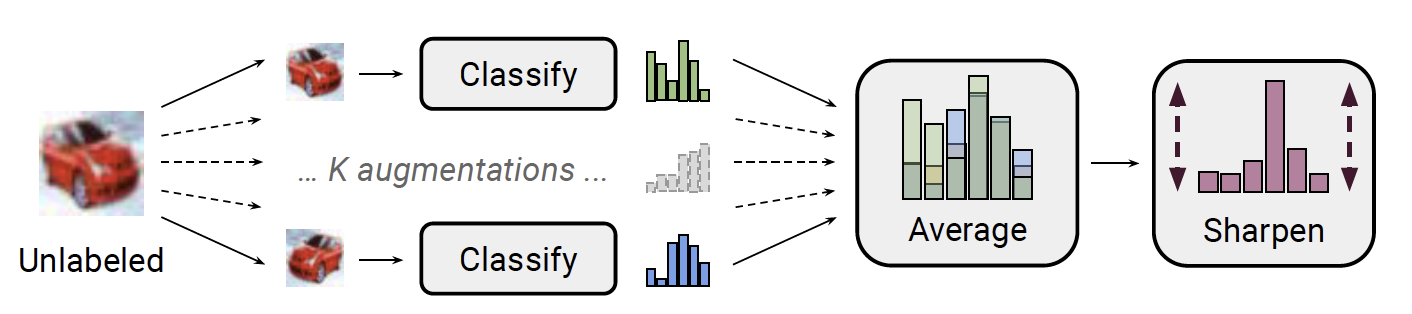MixMatch16 方法如下:

1. 数据增广. 有标签数据 $$(x_b, p_b)\in\mathcal{D}_l, b \in (1,\dots,B)$$, 其中 $$B$$ 为 batch size, 计算其增广 $$\hat{x}_b$$. 无标签数据 $$u_b\in\mathcal{D}_u, b \in (1,\dots,B)$$, 计算其 $$K$$ 个增广 $$\hat{u}_{b,1},\dots,\hat{u}_{b,K}$$.
2. 打伪标签. 这一步是给无标签数据打伪标签. 使用模型 $$f_{\theta}$$ 对 $$K$$ 个增广数据进行预测, 取平均预测结果作为 $$K$$ 个增广数据共同的伪标签

$q_b = \frac1K\sum_{k=1}^K f_{\theta}(\hat{u}_{b,k})$
3. 锐化. 通过调整温度系数 $$T$$, 对上一步的伪标签结果进行锐化

$\text{Sharpen}(q, T) = q_i^{\frac1T} \Big/ \sum_{j=1}^C q_j^{\frac1T}$

其中 $$C$$ 是类别数.

4. MixUp. 在一个 batch 中, 对增广的有标签数据和增广的伪标签数据

\begin{align} \hat{\mathcal{X}} &= \{(\hat{x}_b, p_b); b \in (1,\dots, B)\} \\ \hat{\mathcal{U}} &= \{(\hat{u}_{b,k}, q_b); b \in (1,\dots,B), k \in (1,\dots,K) \} \end{align}

进行打乱得到混合数据 $$\mathcal{W}=\text{Shuffle}(\text{Concat}(\hat{\mathcal{X}}, \hat{\mathcal{U}}))$$. 然后再随机划分为和集合 $$\hat{\mathcal{X}}, \hat{\mathcal{U}}$$ 一样大小的 $$\hat{\mathcal{W}}_1, \hat{\mathcal{W}}_2$$. 接着使用 MixUp 生成新的增广数据:

\begin{align} \mathcal{X}' &= \text{MixUp}(\hat{\mathcal{X}}, \hat{\mathcal{W}}_1) \\ \mathcal{U}' &= \text{MixUp}(\hat{\mathcal{U}}, \hat{\mathcal{W}}_2) \end{align}

其中在使用 MixUp 时保证左侧集合占主导($$\eqref{eq:mixup_lambda}$$ 式), 即混合数据靠近左侧的数据. MixUp 方式如下:

\begin{align} \lambda &\sim \text{Beta}(\alpha, \alpha) \\ \label{eq:mixup_lambda} \lambda' &= \max(\lambda, 1-\lambda) \\ x' &= \lambda'x_1 + (1 - \lambda')x_2 \\ p' &= \lambda'p_1 + (1 - \lambda')p_2 \end{align}

\begin{align} \mathcal{L} &= \mathcal{L}_s + w\mathcal{L}_u \\ &= \frac1{\vert\mathcal{X}'\vert}\sum_{x,p\in\mathcal{X}'}H(p, f_{\theta}(x)) + w\frac1{\vert\mathcal{U}'\vert}\sum_{u,q\in\mathcal{U}'}\Vert q-f_{\theta}(u)\Vert_2^2 \end{align}

### 5.2 ReMixMatch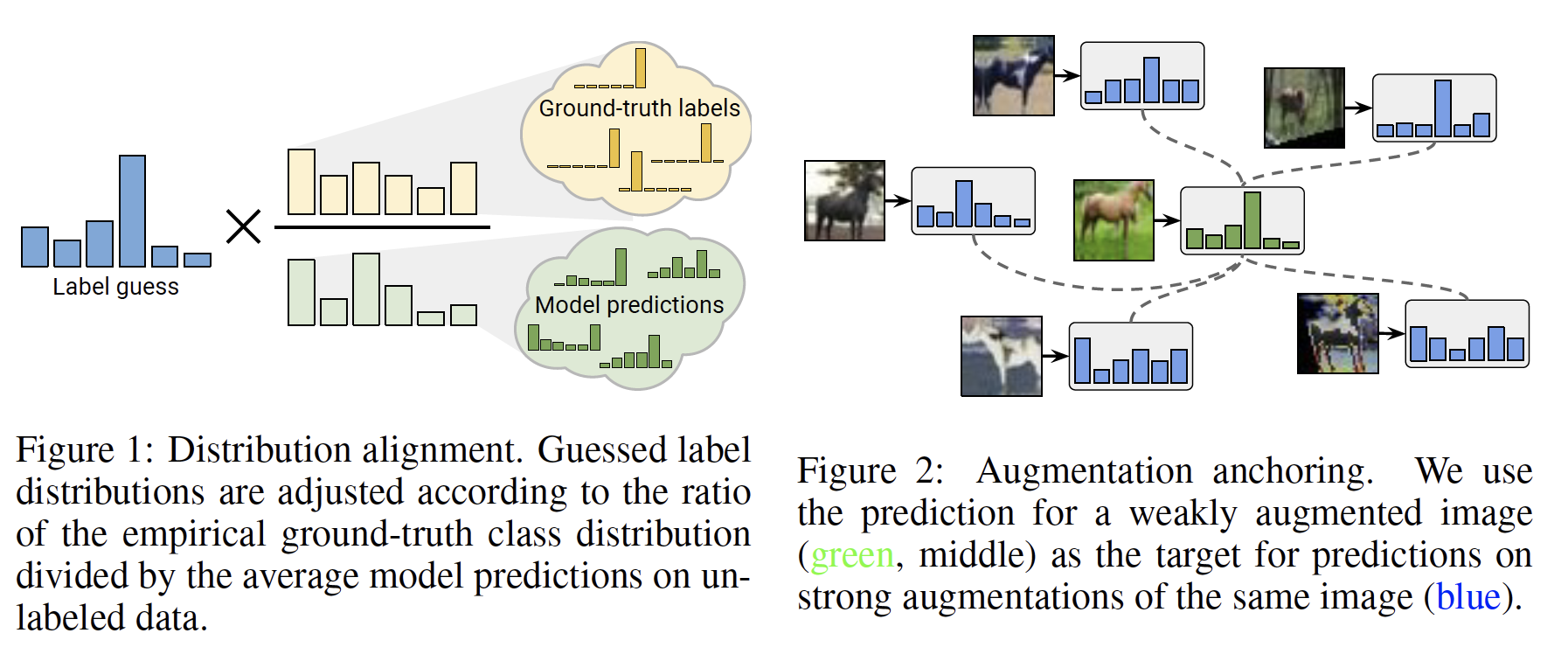ReMixMatch17 在 MixMatch 的基础上, 引入了两个新技术:

• 分布对齐 (distribution alignment): 要求无标签数据的预测(边缘)分布要对齐有标签数据的(边缘)分布
• 增广锚定 (augmentation anchoring): 要求多个强增广数据的预测接近弱增广数据的预测 (锚点).

### 5.3 FixMatch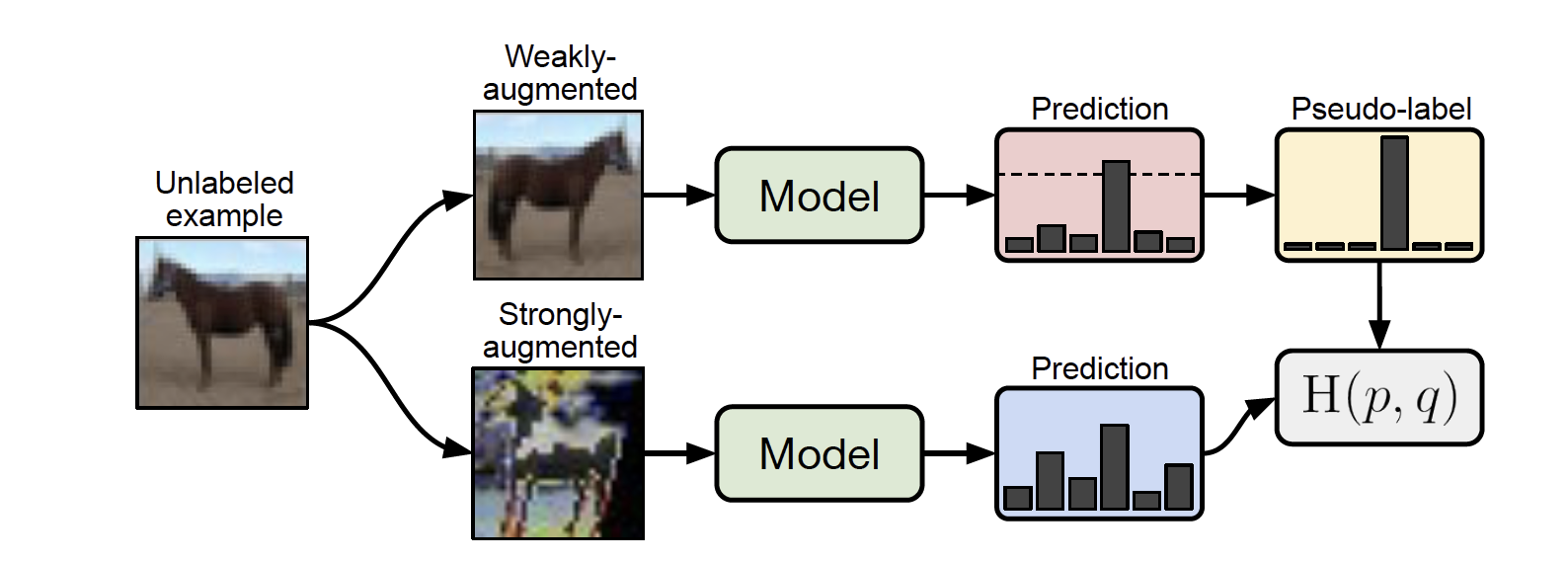FixMatch18: 对于无标签数据, 如果模型 weakly-augmentated 样本的预测类别的置信度大于一个阈值, 则该类别当做伪标签用于训练 strongly-augmentated 的样本.

$\mathcal{L}_u = \frac1{K\vert\mathcal{D}_u\vert}\sum_{x\in\mathcal{D}_u}\sum_{i=1}^K\mathbf{1}(\max(f_{\theta}(A_w(x))) \geq \tau)H(f_{\theta}(A_w(x)), f_{\theta}(A_s(x)))$

## 参考文献

1. Big Self-Supervised Models are Strong Semi-Supervised Learners
T. Chen, S. Kornblith, K. Swersky, M. Norouzi, and G. Hinton
[pdf] In NeurIPS 2020

2. Semi-Supervised Learning (Book)
Chapelle O., Scholkopf B., Zien A. Eds
[html] In IEEE Transactions on Neural Networks 20, 542–542 (2009)

3. On Data-Augmentation and Consistency-Based Semi-Supervised Learning
Ghosh A., Thiery A. H.
[html] In ICLR 2021  2

4. Semi-Supervised Learning with Ladder Networks
Rasmus A., Valpola H., Honkala M., Berglund M., Raiko, T.
[html] In arXiv:1507.02672

5. Temporal Ensembling for Semi-Supervised Learning
S. Laine and T. Aila
[html] In arXiv:1610.02242  2

6. Mean teachers are better role models: Weight-averaged consistency targets improve semi-supervised deep learning results
A. Tarvainen and H. Valpola
[pdf] In NeurIPS 2017

7. Dual Student: Breaking the Limits of the Teacher in Semi-Supervised Learning
Z. Ke, D. Wang, Q. Yan, J. Ren, and R. W. H. Lau
[html] In CVPR 2019

8. MixUp: Beyond Empirical Risk Minimization
H. Zhang, M. Cisse, Y. N. Dauphin, and D. Lopez-Paz
[html] In ICLR 2018

9. AutoAugment: Learning Augmentation Policies from Data
E. D. Cubuk, B. Zoph, D. Mane, V. Vasudevan, and Q. V. Le
[html] In CVPR 2019

10. RandAugment: Practical automated data augmentation with a reduced search space
E. D. Cubuk, B. Zoph, J. Shlens, and Q. Le
[pdf] In NeurIPS 2020

11. Pseudo-Labeling and Confirmation Bias in Deep Semi-Supervised Learning
E. Arazo, D. Ortego, P. Albert, N. E. O’Connor, and K. McGuinness
[html] In IJCNN 2020

12. Label Propagation for Deep Semi-Supervised Learning
A. Iscen, G. Tolias, Y. Avrithis, and O. Chum
[html] In CVPR 2019

13. Learning from Labeled and Unlabeled Data with Label Propagation
X. Zhu and Z. Ghahramani
[pdf] In 2002

14. Combining labeled and unlabeled data with co-training
A. Blum and T. Mitchell
[html] In COLT 1998

15. Tri-training: exploiting unlabeled data using three classifiers
Zhi-Hua Zhou; Ming Li
[html] In TKDE 2005

16. MixMatch: A holistic approach to semi-supervised learning
D. Berthelot, N. Carlini, I. Goodfellow, N. Papernot, A. Oliver, and C. A. Raffel
[pdf] In NeurIPS 2019

17. ReMixMatch: Semi-Supervised Learning with Distribution Matching and Augmentation Anchoring
D. Berthelot
[html] In ICLR 2019

18. FixMatch: Simplifying semi-supervised learning with consistency and confidence
K. Sohn
[pdf] In NeurIPS 2020

Content